Get inspired by the success stories of our students in IIT JAM MS, ISI  MStat, CMI MSc DS.  Learn More

# ISI MStat PSB 2014 Problem 9 | Hypothesis TestingThis is a another beautiful sample problem from ISI MStat PSB 2014 Problem 9. It is based on testing simple hypothesis, but reveals and uses a very cute property of Geometric distribution, which I prefer calling sister to Loss of memory . Give it a try !

## Problem- ISI MStat PSB 2014 Problem 9

Let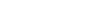and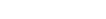be independent random variables, where Geo(p) refers to Geometric distribution whose p.m.f. f is given by,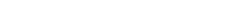We are interested in testing the null hypothesis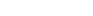against the alternative. Intuitively it is clear that we should reject if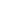is large, but unfortunately, we cannot compute the cut-off because the distribution ofunder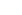depends on the unknown (common) value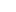and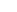.

(a) Let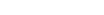. Find the conditional distribution of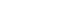when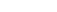.

(b) Based on the result obtained in (a), derive a level 0.05 test foragainstwhenis large.

### Prerequisites

Geometric Distribution.

Negative binomial distribution.

Discrete Uniform distribution .

Conditional Distribution . .

Simple Hypothesis Testing.

## Solution :

Well, Part (a), is quite easy, but interesting and elegant, so I'm leaving it as an exercise, for you to have the fun. Hint: verify whether the required distribution is Discrete uniform or not ! If you are done, proceed .

Now, part (b), is further interesting, because here we will not use the conventional way of analyzing the distribution ofand, whereas we will be concentrating ourselves on the conditional distribution of! But why ?

The reason behind this adaptation of strategy is required, one of the reason is already given in the question itself, but the other reason is more interesting to observe , i.e. if you are done with (a), then by now you found that , the conditional distribution ofis independent of any parameter ( i.e. ithe distribution oflooses all the information about the parameter, when conditioned by Y=y ,is a necessary condition), and the parameter independent conditional distribution is nothing but a Discrete Uniform {0,1,....,y}, where y is the sum ofand.

so, under, the distribution ofis independent of the both common parameterand. And clearly as stated in the problem itself, its intuitively understandable , large value ofexhibits evidences against. Since large value ofis realized, means the success doesn't come very often .i.e.is smaller.

So, there will be strong evidence againstif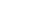, where , for some constant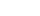, where y is given the sum of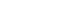.

So, for a level 0.05 test , the test will rejectfor large value of k , such that,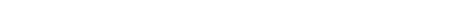So, we rejectat level 0.05, when we observe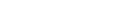, where it is given that=y . That's it!

## Food For Thought

Can you show that for this sameand,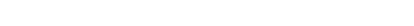considering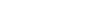, where n=0,1,.... What about the converse? Does it hold? Find out!

But avoid loosing memory, it's beauty is exclusively for Geometric ( and exponential) !!

## Subscribe to Cheenta at Youtube

This is a another beautiful sample problem from ISI MStat PSB 2014 Problem 9. It is based on testing simple hypothesis, but reveals and uses a very cute property of Geometric distribution, which I prefer calling sister to Loss of memory . Give it a try !

## Problem- ISI MStat PSB 2014 Problem 9

Letandbe independent random variables, where Geo(p) refers to Geometric distribution whose p.m.f. f is given by,We are interested in testing the null hypothesisagainst the alternative. Intuitively it is clear that we should reject ifis large, but unfortunately, we cannot compute the cut-off because the distribution ofunderdepends on the unknown (common) valueand.

(a) Let. Find the conditional distribution ofwhen.

(b) Based on the result obtained in (a), derive a level 0.05 test foragainstwhenis large.

### Prerequisites

Geometric Distribution.

Negative binomial distribution.

Discrete Uniform distribution .

Conditional Distribution . .

Simple Hypothesis Testing.

## Solution :

Well, Part (a), is quite easy, but interesting and elegant, so I'm leaving it as an exercise, for you to have the fun. Hint: verify whether the required distribution is Discrete uniform or not ! If you are done, proceed .

Now, part (b), is further interesting, because here we will not use the conventional way of analyzing the distribution ofand, whereas we will be concentrating ourselves on the conditional distribution of! But why ?

The reason behind this adaptation of strategy is required, one of the reason is already given in the question itself, but the other reason is more interesting to observe , i.e. if you are done with (a), then by now you found that , the conditional distribution ofis independent of any parameter ( i.e. ithe distribution oflooses all the information about the parameter, when conditioned by Y=y ,is a necessary condition), and the parameter independent conditional distribution is nothing but a Discrete Uniform {0,1,....,y}, where y is the sum ofand.

so, under, the distribution ofis independent of the both common parameterand. And clearly as stated in the problem itself, its intuitively understandable , large value ofexhibits evidences against. Since large value ofis realized, means the success doesn't come very often .i.e.is smaller.

So, there will be strong evidence againstif, where , for some constant, where y is given the sum of.

So, for a level 0.05 test , the test will rejectfor large value of k , such that,So, we rejectat level 0.05, when we observe, where it is given that=y . That's it!

## Food For Thought

Can you show that for this sameand,considering, where n=0,1,.... What about the converse? Does it hold? Find out!

But avoid loosing memory, it's beauty is exclusively for Geometric ( and exponential) !!

## Subscribe to Cheenta at Youtube

This site uses Akismet to reduce spam. Learn how your comment data is processed.

### Knowledge Partner